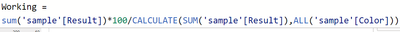cancel
Showing results for
Did you mean:Regular Visitor

## Percentage of text values

Hi,

I need to find the percentage of survey having rounds "pilot" and "1" with values Green, Red and Yellow.  for example, in Round "Pilot" - Green = 200, Yellow - 100, Red = 36 and In round "1" - Green =400, Yellow =130, Red = 40. Result should be like for Round Pilot - Green = 60%, Yellow = 30%, Red = 10%

Round "1" -  Green = 70%, Yellow = 23%, Red = 7%

need to represent this data in a line graph.

2 REPLIES 2Super User

Hi @hhari

If I understood correctly, your sample is like below and you need to see result 'working' as below:Possibly you can use formula as below:Here in the visual we are using two columns, Round and color, so as a row context we are filtering individual values and then in denominator we are removing filter of all colors.

Hope it helps!Regular Visitor

Apologies for the inaccurate information. Kindly refer to the provided screenshot displaying the raw data. Our objective is to present this data in the form of a line graph, as shown below. We aim to determine the frequency of values in the raw data and visualize it using a line graph. Your assistance in this matter would be greatly appreciated.

Raw dataResult